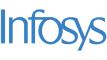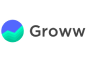New update is available. Click here to update.
Topics

# Find the Good Matrix

Moderate0/80
Average time to solve is 30m+2 more companies

## Problem statement

You have given a 2-dimensional array ‘ARR’ with ‘N’ rows and ‘M’ columns in which each element contains only two values,i.e., 0 and 1. Your task is to convert the given matrix into the Good matrix in which if an element is 0, you need to set all elements values present in its entire row and column to 0.

For example:

``````Consider ARR = [[1 , 0 , 1] ,
[1 , 1 , 1] ,
[1 , 1 , 1]],
the Good matrix after updating the given matrix as described in the question is
[[0 , 0 , 0] ,
[1 , 0 , 1] ,
[1 , 0 , 1]].
Since ARR is 0, we need to set all element’s values present in 0-th row and 1-th column to 0.
``````

Note :

``````You do not need to print the matrix. Just change in the given input.
``````
Detailed explanation ( Input/output format, Notes, Images )
Constraints :
``````1 <= T <= 20
1 <= N <= 300
1 <= M <= 300

ARR[i][j] can only contain two values, i.e, 0 and 1.

Where 'T' denotes the number of test cases, 'N' and 'M' denotes the number of rows and the number of columns in the array ‘ARR’ respectively, and 'ARR[i][j]' denotes the ’j-th’ element of  'i-th' row of the array 'ARR'.

Time Limit: 1sec
``````
Sample Input 1 :
``````2
2 2
0 1
1 1
3 3
1 1 0
1 1 1
1 1 1
``````
Sample Output 1 :
``````0 0
0 1
0 0 0
1 1 0
1 1 0
``````
Explanation of sample input 1:
``````For the first test case,
The Good matrix after updating the given matrix as described in the question is
[[0 , 0] ,
[0 , 1]].
Since ARR is 0, we need to set all elements value present in 0-th row and 0-th column to 0.

For the second test case,
The Good matrix after updating the given matrix as described in the question is
[[0 , 0 , 0] ,
[1 , 1 , 0] ,
[1 , 1 , 0]].
Since ARR is 0, we need to set all elements value present in 0-th row and 2-th column to 0.
``````
Sample Input 2 :
``````2
4 4
1 1 1 1
0 1 1 1
1 1 1 1
0 1 1 1
3 3
0 1 1
0 1 1
1 1 1
``````
Sample Output 2 :
``````0 1 1 1
0 0 0 0
0 1 1 1
0 0 0 0
0 0 0
0 0 0
0 1 1
``````Console